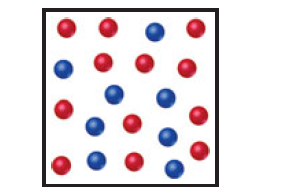×
Get Full Access to Chemistry: The Central Science - 12 Edition - Chapter 2 - Problem 2e
Get Full Access to Chemistry: The Central Science - 12 Edition - Chapter 2 - Problem 2e

×

# Solved: Visualizing ConceptsThese exercises are intendedISBN: 9780321696724 27

## Solution for problem 2E Chapter 2

Chemistry: The Central Science | 12th Edition

• Textbook Solutions
• 2901 Step-by-step solutions solved by professors and subject experts
• Get 24/7 help from StudySoup virtual teaching assistantsChemistry: The Central Science | 12th Edition

4 5 1 400 Reviews
27
5
Problem 2E

The following diagram is a representation of 20 atoms of a fictitious element, which we will call nevadium (Nv). The red spheres are 293Nv, and the blue spheres are 295Nv. (a) Assuming that this sample is a statistically representative sample of the element, calculate the percent abundance of each element. (b) If the mass of 293Nv is 293.15 amu and that of 295Nv is 295.15 amu, what is the atomic weight of Nv? [Section 2.4]Step-by-Step Solution:
Step 1 of 3

Monday, February 1, 2016 Chapter 2 cont. charge on e = 1.609 x 10 -19c calculated mass on e = 9.11 x 10 -31kg Millikan Oil Drop experiment - Observed charges on oil droplets produced by x-rays with voltage on and voltage off. Voltage on-> droplets with negative charge will fall more slowly or even rise. - There must be another particle to neutralize atom. Proton • Radioactive material discoveries - gamma γ: high energy photons - alpha α: (helium nucleus) heavy emission - beta β: (free electrons) negative emission • Rutherford (1871-1937) Gold Foil experiment - tests “plum-pudding” model and concludes with his “nuclear” model - Noble Prize in Chemistry 1908 α Bombardment of Metal Foil: about 1

Step 2 of 3

Step 3 of 3

##### ISBN: 9780321696724

Unlock Textbook Solution